﻿ 一种小型频率选择表面结构设计方法
 舰船科学技术2016, Vol. 38Issue (6): 137-140PDF

1. 海军驻沪东中华造船(集团)有限公司军事代表室, 上海 200129 ;
2. 中国舰船研究设计中心, 湖北 武汉 430064

Novel design method of frequency selective surface
XIAO Wei-dong1, XIONG Bo2, DENG Feng2, CHEN Liang2
1. Martial Commissary Quarterat Hudong-Zhonghua Shipbuilding (group) Co., Ltd., Shanghai 200129, China ;
2. China Ship Research and Design Center, Wuhan 430064, China
Abstract: This paper proposed a novel method to design the structure of FSS which based on the principle of polyhedral mutual coupling. The equivalent model can be obtained through the theory, thus, we can calculate the characteristics of the FSS according to the model. The relationship between the structure of FSS and the parameters of the equivalent model with the method. Then, we can calculate the parameters of the structure directly with the given characteristics of the FSS.
Key words: transmission line equal model     FSS     periodic structure
0 引 言

1 等效模型方法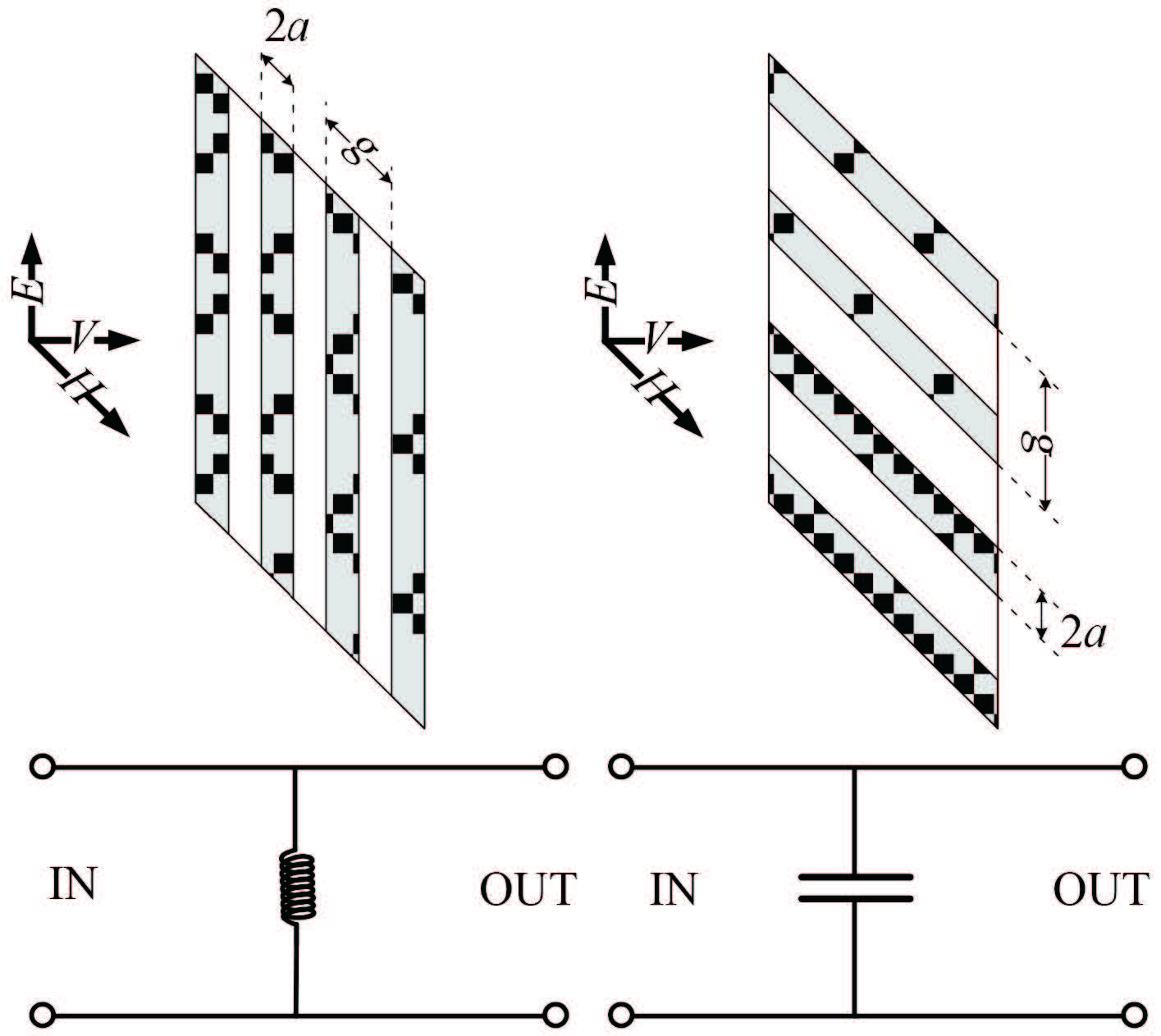图 1 不同极化方式下周期性长带线的等效集总参数模型 Fig. 1 The equivalent lumped parameter model of periodic strapline under different polarization

 $\begin{array}{l} {X_{TE}}{ = ^{w.L}}{/_{{Z_o}}} = \\[10pt] \displaystyle\frac{{p.\cos (\theta )}}{\lambda }.({\rm ln}({\rm cosec}\pi w/2p)) + G(p,w,\lambda ,\theta ))\text{；} \end{array}$ (1)

 $\begin{array}{l} {B_{TE}}{ = ^{w.C}}{/_{{Y_o}}} = \\[10pt] \displaystyle\frac{{4.p.\sec (\theta )}}{\lambda }.({\rm ln}({\rm cosec}(\pi g/2p)) + G(p,w,\lambda ,\theta ))\text{。} \end{array}$ (2)

 $\begin{array}{l}\textstyle G(p,w,\lambda ,\theta ) = \frac{{0.5{{(1 - {\beta ^2})}^2}\left[{\left( {1 - \frac{{{\beta ^2}}}{4}} \right)({A_{1 + }} + {A_{1 - }}) + 4{\beta ^2}{A_{1 + }}{A_{1 - }}} \right]}}{{\left( {1 - \frac{{{\beta ^2}}}{4}} \right) + {\beta ^2}\left( {1 + \frac{{{\beta ^2}}}{2} - \frac{{{\beta ^4}}}{8}} \right)({A_{1 + }} + {A_{1 - }}) + 2{\beta ^6}{A_{1 + }}{A_{1 - }}}}\\[5pt] \textstyle {A_{1 \pm }} = \frac{1}{{\sqrt {{{\left( {\frac{{p\sin \theta }}{\lambda } \pm 1} \right)}^2} - \frac{{{p^2}}}{{{\lambda ^2}}}} }} - 1 \\ \beta = \sin (0.5\pi w/p)\text{。} \end{array}$ (3)

2 小型化周期单元的等效模型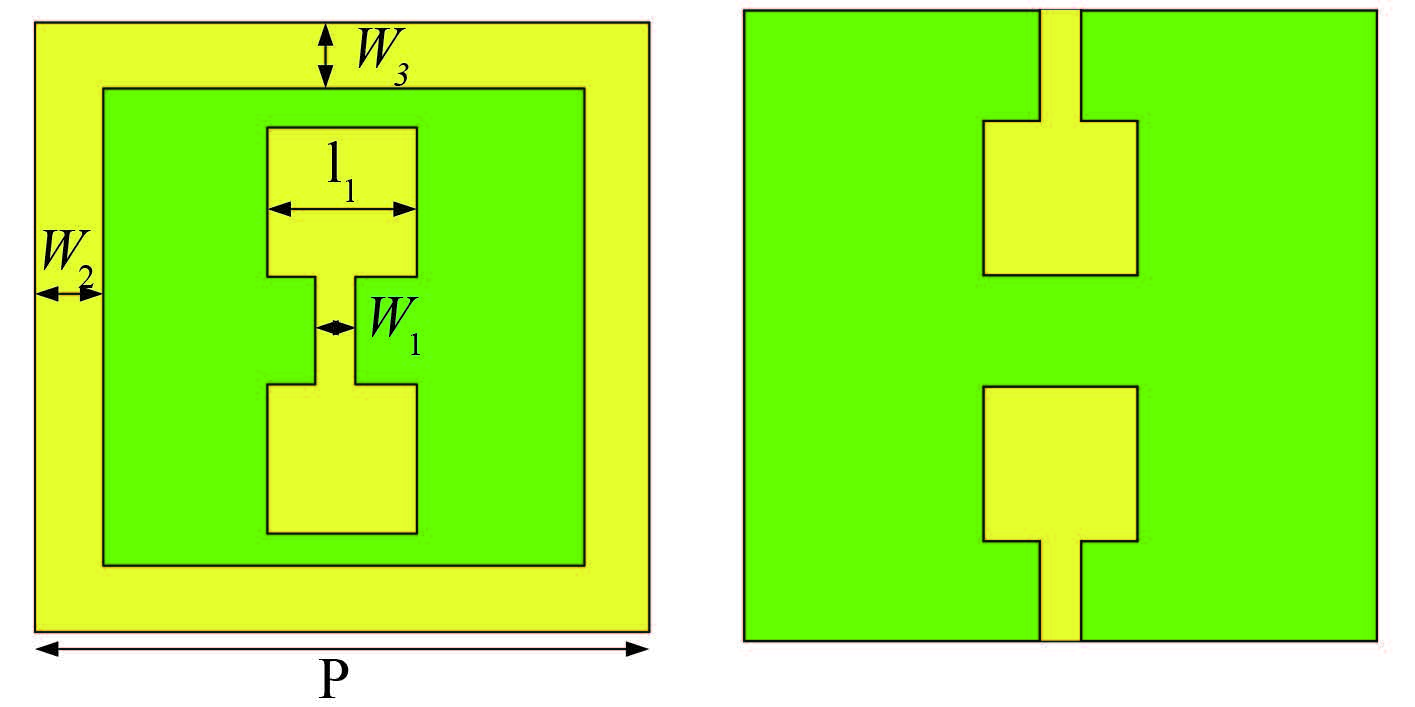图 2 一种基于多面互耦合原理的小型化频率选择表面周期单元结构 Fig. 2 Miniaturization frequency selective surface cycle unit structure based on the principle of polyhedral mutual coupling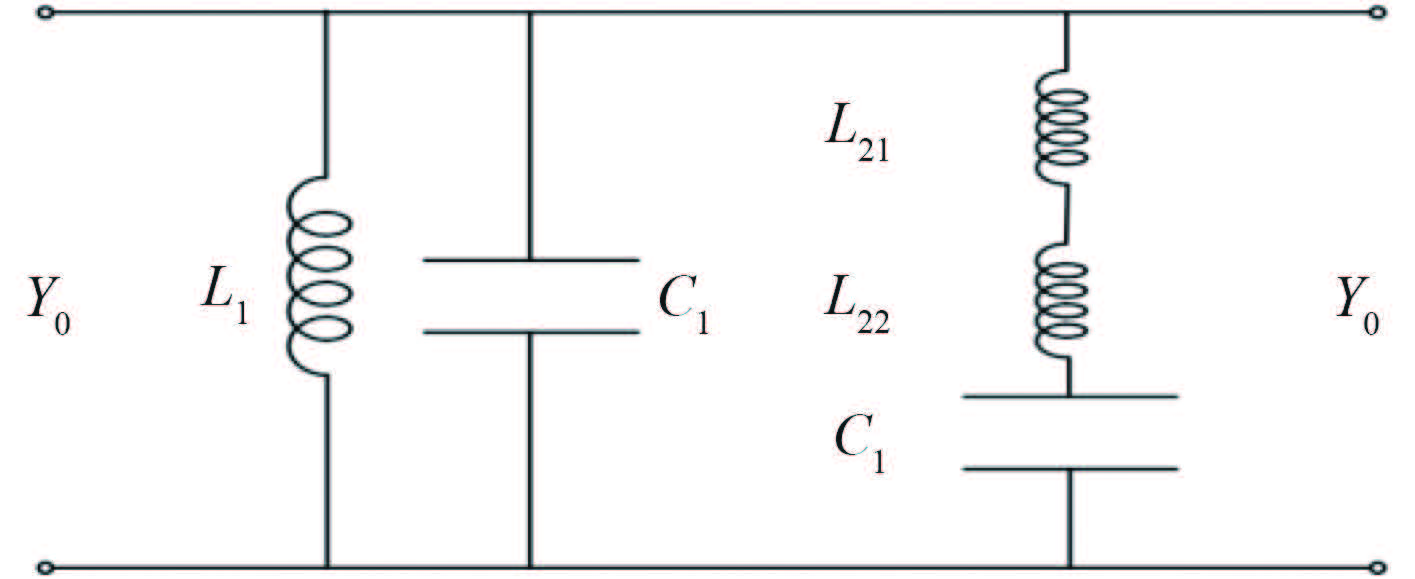图 3 小型化周期单元的传输线等效模型 Fig. 3 The equivalent transmission line model of miniaturization cycle unit structure

 $\begin{array}{l} {L_1} = \displaystyle\frac{{P - 2{w_3}}}{P} \cdot \displaystyle\frac{{\lambda \cdot F\left( {P,2{w_2},\lambda } \right) \cdot {Z_0}}}{{2\pi \cdot C}}\text{，}\\ {L_{21}} = \displaystyle\frac{{P - 2{w_3} - 2{l_1}}}{P} \cdot \displaystyle\frac{{\lambda \cdot F\left( {P,{w_1},\lambda } \right) \cdot {Z_0}}}{{2\pi \cdot C}}\text{，}\\ {L_{22}} = \displaystyle\frac{{2{l_1}}}{P} \cdot \displaystyle\frac{{\lambda \cdot F\left( {P,{l_1},\lambda } \right) \cdot {Z_0}}}{{2\pi \cdot C}}\text{，}\\ {C_1} = \displaystyle\frac{{P - 2{w_2}}}{{2P}} \cdot \displaystyle\frac{{\lambda \cdot F\left( {P,P - 2{w_3},\lambda } \right)}}{{2\pi \cdot C \cdot {Z_0}}}\text{，}\\ {C_2} = \displaystyle\frac{{l_1^2 \cdot {\varepsilon _0} \cdot {\varepsilon _r}}}{{2 \cdot h}}\text{。} \end{array}$ (4)

 $F(P,{D_1},\lambda) = \frac{P}{\lambda } \cdot \cos d(\theta) \cdot (\text{log}\left( {\frac{1}{{\sin \left( {\frac{{\pi \cdot D}}{{2P}}} \right)}}} \right) + G)\text{。}$ (5)

 $\textstyle {f_0} = \frac{{\sqrt {(({L_1} \cdot {C_2} + {L_2} \cdot {C_2} + {L_1} \cdot {C_1} - \sqrt {C_2^2 \cdot {{\left( {{L_1} + {L_2}} \right)}^2} + L_1^2 \cdot C_1^2 + 2{C_1}{C_2}{L_1}\left( {{L_1} - {L_2}} \right)} } }}{{2{C_1}{C_2}{L_1}{L_2}}}\text{。}$ (6)
3 等效模型验证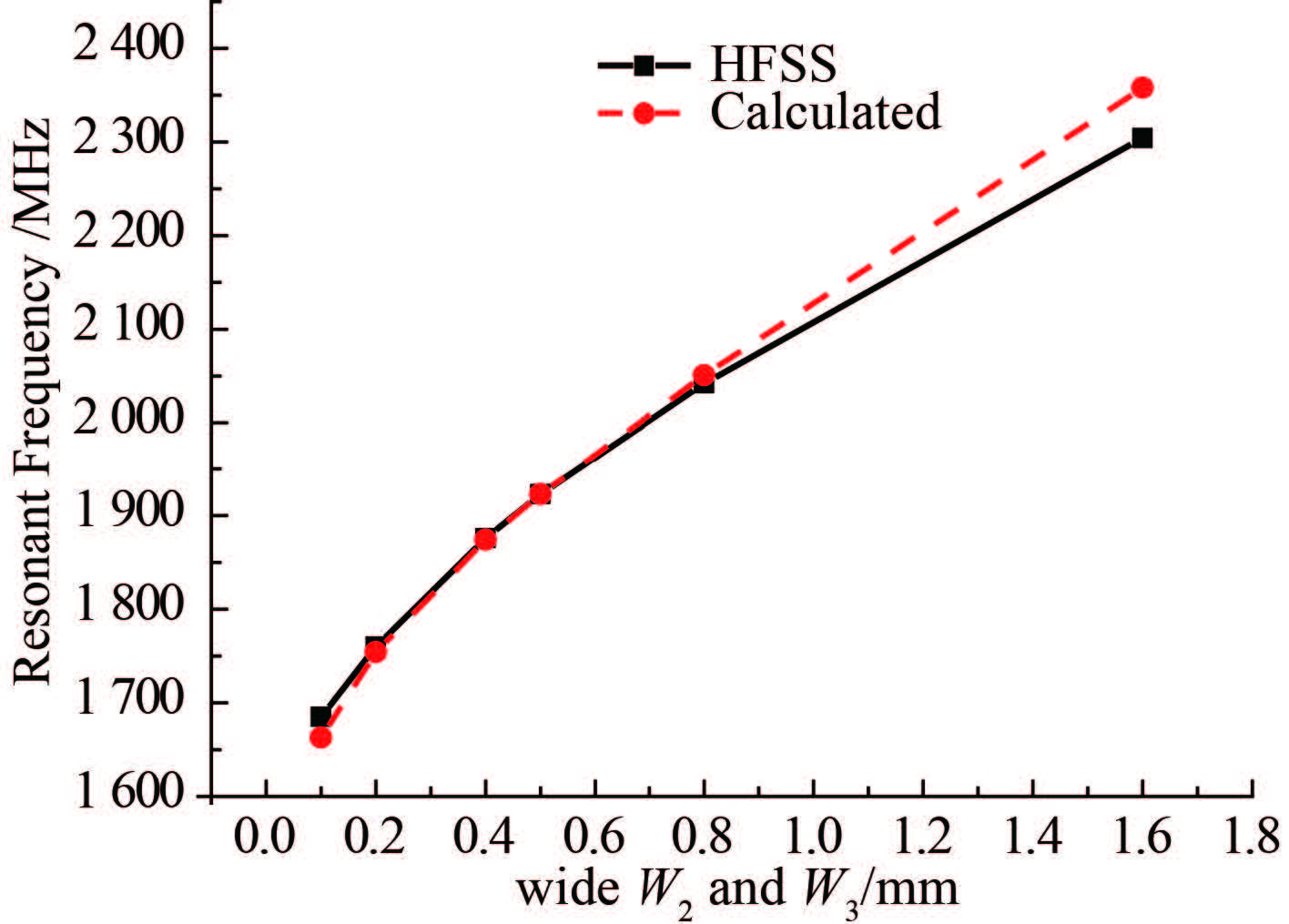图 4 W2 和 W3 变化时，全波仿真及等效电路模型计算结果对比 Fig. 4 Result of simulation and equivalent circuit model while the W2 and W3 is variable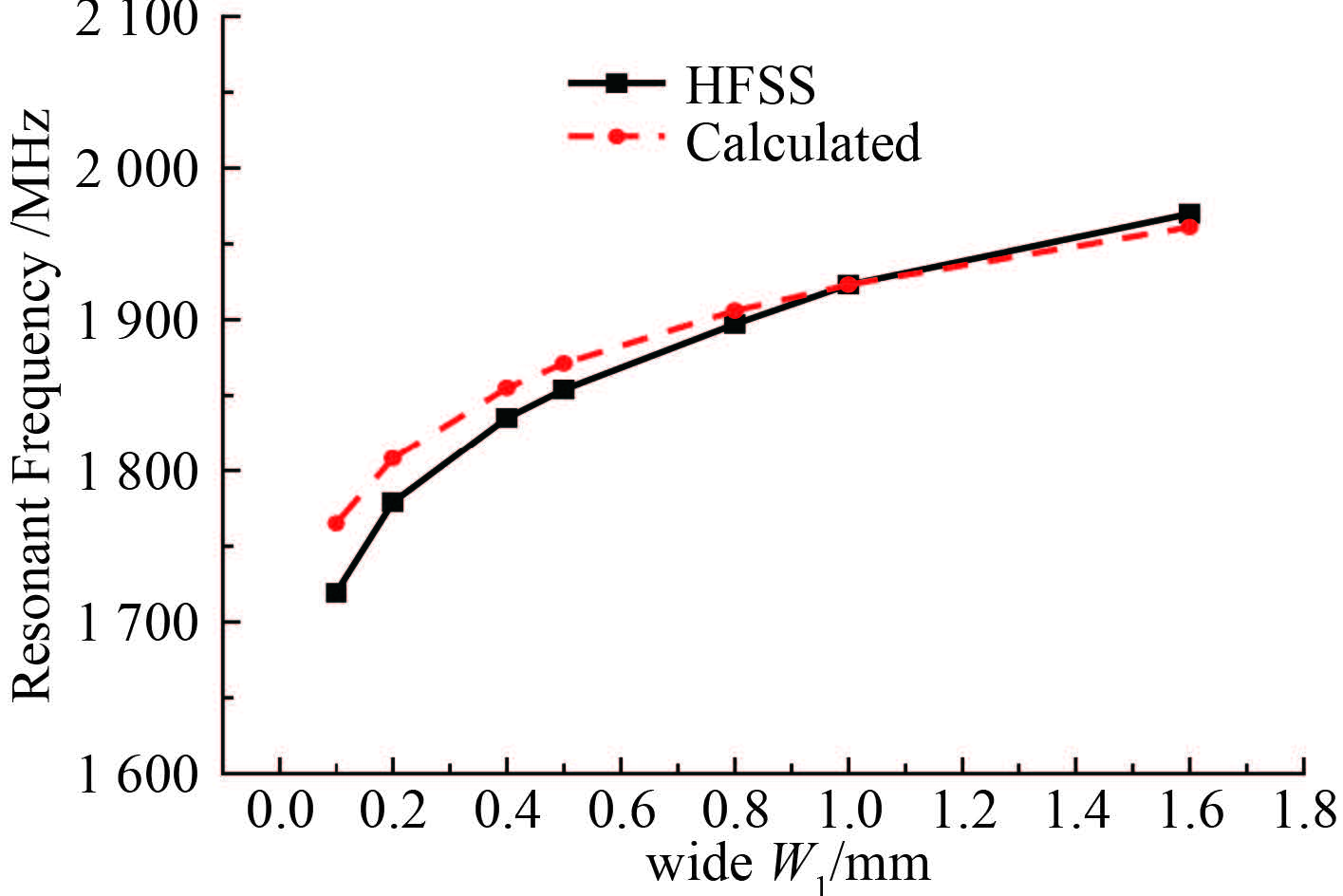图 5 W1变化时，全波仿真及等效电路模型计算结果对比 Fig. 5 Result of simulation and equivalent circuit model while the W1 is variable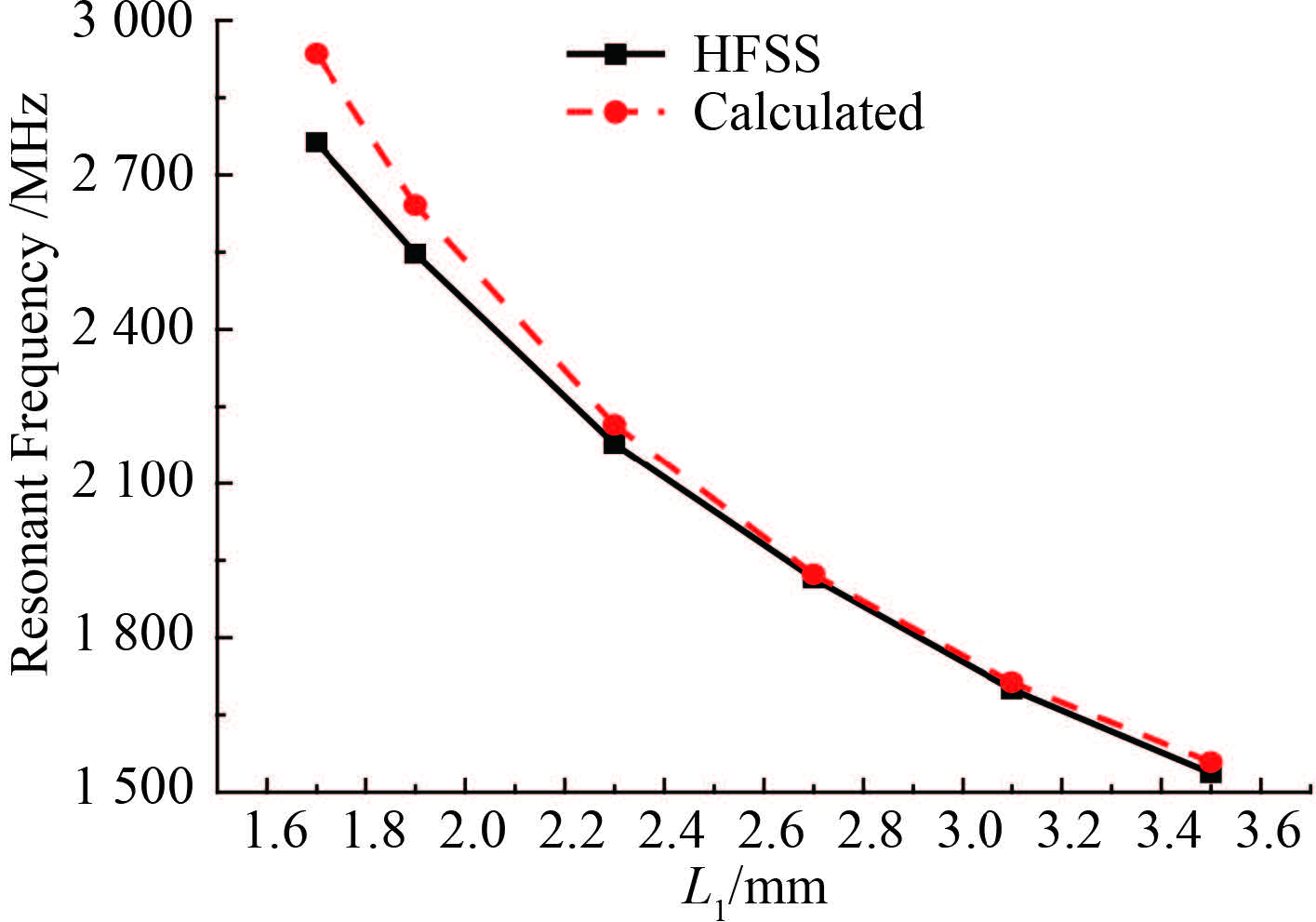图 6 L1变化时，全波仿真及等效电路模型计算结果对比 Fig. 6 Result of simulation and equivalent circuit model while the L1 is variable
4 结 语

  MUNK B A. Frequency selective surfaces-theory and design[M]. New York: John Wiley and Sons Inc, 2000 .  MITTRA R, CHAN C H, CWIK T. Techniques for analyzing frequency selective surfaces-a review[J]. Proceedings of the IEEE , 1988, 76 (12) :1593–1615. DOI:10.1109/5.16352  BARDI I, REMSKI R, PERRY D, et al. Plane wave scattering from frequency-selective surfaces by the finite-element method[J]. IEEE Transactions on Magnetics , 2002, 38 (2) :641–644. DOI:10.1109/20.996167  LANGLEY R J, PARKER E A. Equivalent circuit model for arrays of square loops[J]. Electronics Letters , 1982, 18 (7) :294–296. DOI:10.1049/el:19820201  LEE C K, LANGLEY R J. Equivalent-circuit models for frequency-selective surfaces at oblique angles of incidence[J]. IEEE Proceedings H-microwaves, Antennas and Propagation , 1985, 132 (6) :395–399. DOI:10.1049/ip-h-2.1985.0070  LI Z, PAPALAMBROS P Y, VOLAKIS J L. Frequency selective surface design by integrating optimisation algorithms with fast full wave numerical methods[J]. IEE Proceedings-microwaves, Antennas and Propagation , 2002, 149 (3) :175–180. DOI:10.1049/ip-map:20020357  RAHMAT-SAMⅡ Y, MICHIELSSEN E. Electromagnetic optimization by genetic algorithms:wiley series in microwave and optical engineering[M]. Hoboken, NJ, USA: Wiley-Interscience, 1999 .  MARCUWITZ N. Waveguide handbook[M]. New York: McGraw-Hill, 1951 .